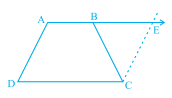"
">

# $\mathrm{ABCD}$ is a trapezium in which $\mathrm{AB} \| \mathrm{CD}$ and $\mathrm{AD}=\mathrm{BC}$ (see below figure). Show that(i) $\angle \mathrm{A}=\angle \mathrm{B}$(ii) $\angle \mathrm{C}=\angle \mathrm{D}$(iii) $\triangle \mathrm{ABC} \equiv \triangle \mathrm{BAD}$(iv) diagonal $\mathrm{AC}=$ diagonal $\mathrm{BD}$[Hint: Extend $\mathrm{AB}$ and draw a line through $\mathrm{C}$ parallel to $\mathrm{DA}$ intersecting $\mathrm{AB}$ produced at E.]"

Given:

$\mathrm{ABCD}$ is a trapezium in which $\mathrm{AB} \| \mathrm{CD}$ and $\mathrm{AD}=\mathrm{BC}$.

To do :

We have to show that

(i) $\angle \mathrm{A}=\angle \mathrm{B}$

(ii) $\angle \mathrm{C}=\angle \mathrm{D}$

(iii) $\triangle \mathrm{ABC} \equiv \triangle \mathrm{BAD}$

(iv) diagonal $\mathrm{AC}=$ diagonal $\mathrm{BD}$

Solution :

Extend $\mathrm{AB}$ and draw a line through $\mathrm{C}$ parallel to $\mathrm{DA}$ intersecting $\mathrm{AB}$ produced at $E$.

(i) $ADCE$ is a parallelogram.

$CE = AD$      (Opposite sides of a parallelogram are equal)

$AD = BC$         (Given)

This implies,

$BC = CE$

$\Rightarrow \angle CBE = \angle CEB$

$\angle EAD+\angle CEB = 180^o$

$\angle EAD+\angle CBE = 180^o$           ($\angle CBE = \angle CEB$)

$\angle CBA +\angle CBE = 180^o$            (Linear pair)

This implies,

$\angle A = \angle B$

(ii) $\angle A+\angle D =180^o$  (Adjacent angles of a parallelogram are supplementary)

$\angle B+\angle C = 180^o$ (Angles on the same side of transversal are supplementary)

$\angle A+\angle D = \angle B+\angle C$

$\angle A+\angle D = \angle A+\angle C$          ($\angle A = \angle B$)

This implies,

$\angle D = \angle C$

(iii) In $\triangle ABC$ and $\triangle BAD$,

$AB = AB$         (Common side)

$\angle DBA = \angle CBA$

$AD = BC$          (Given)

Therefore, by SAS congruency, we get,

$\triangle ABC \cong \triangle BAD$

(iv) $\triangle ABC \cong \triangle BAD$

This implies,

$AC = BD$         (CPCT)

Therefore,

diagonal $\mathrm{AC}=$ diagonal $\mathrm{BD}$.

Updated on: 10-Oct-2022

19 Views